# RD Sharma Solutions for Class 11 Chapter 23 - The Straight Lines Exercise 23.14

This exercise gives an overview of the concept based on the position of two points relative to a line. Students who face difficulty in understanding the concepts during class hours can opt to study RD Sharma Solutions. The experts at BYJU’S have formulated the solutions based on students’ grasping abilities. Students can solve both chapter-wise and exercise wise problems to increase their confidence level, before appearing for the board exam. To boost interest among students in this chapter, RD Sharma Class 11 Maths Solutions Chapter 23 Exercise 23.14, pdf links are given here for easy access.

## Download the pdf of RD Sharma Solutions for Class 11 Maths Exercise 23.14 Chapter 23 – The Straight Lines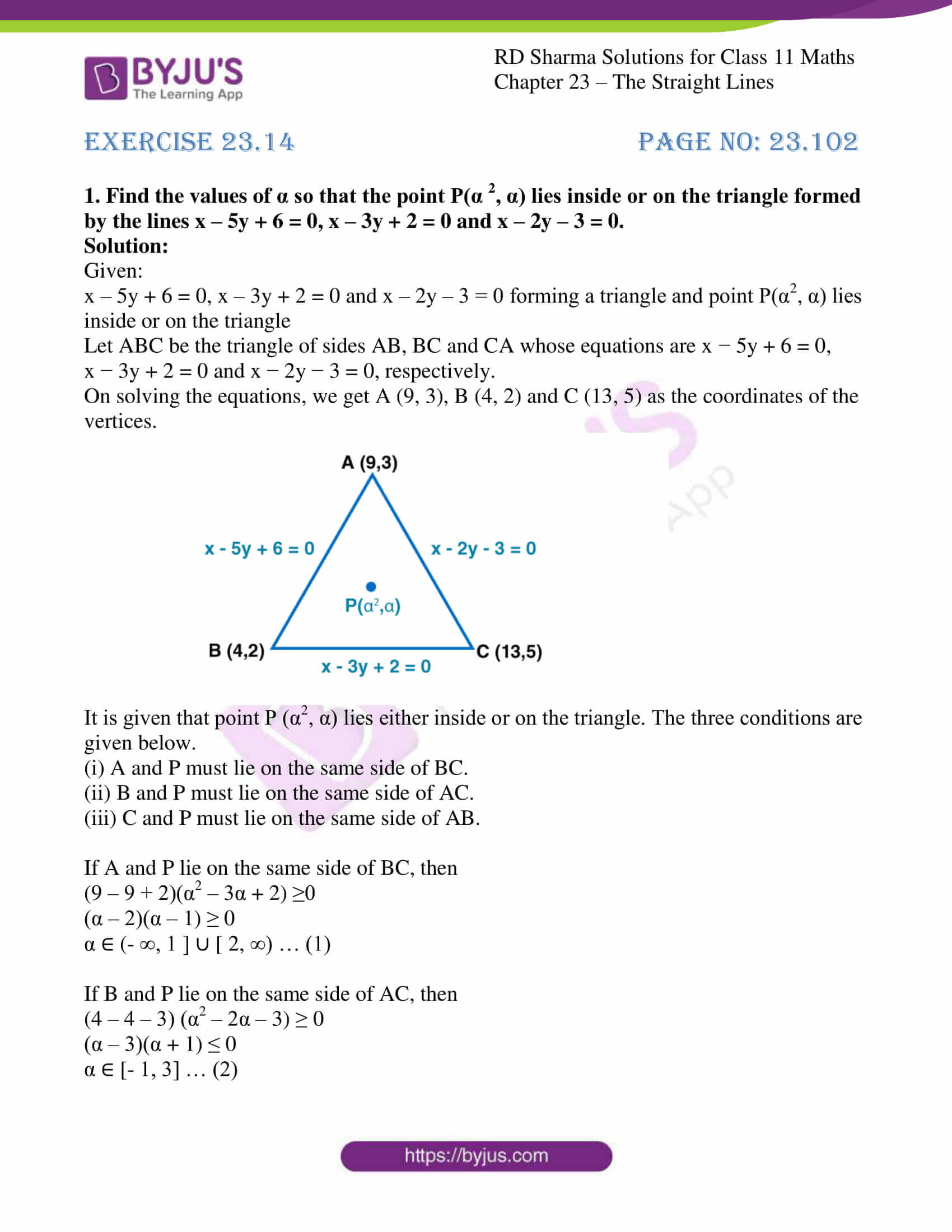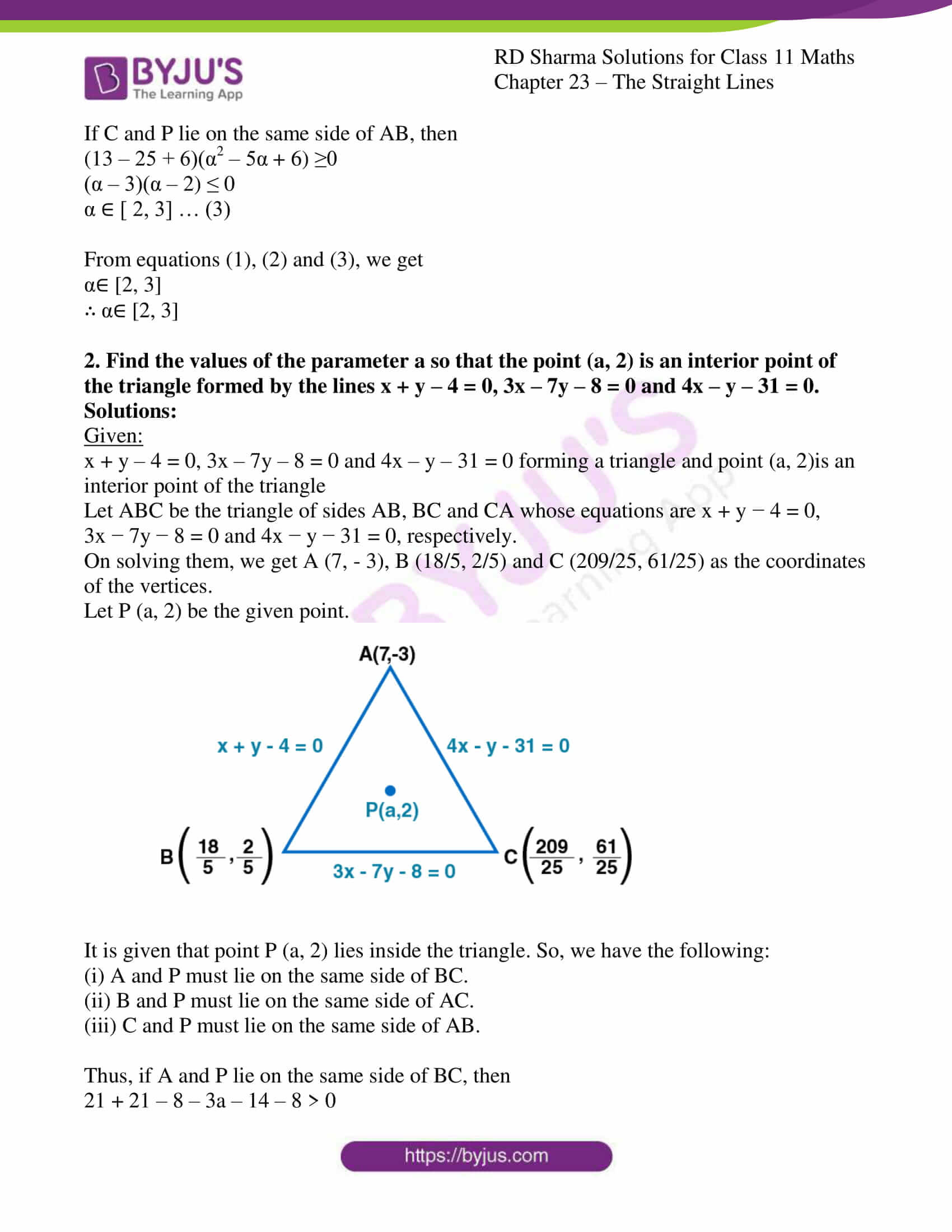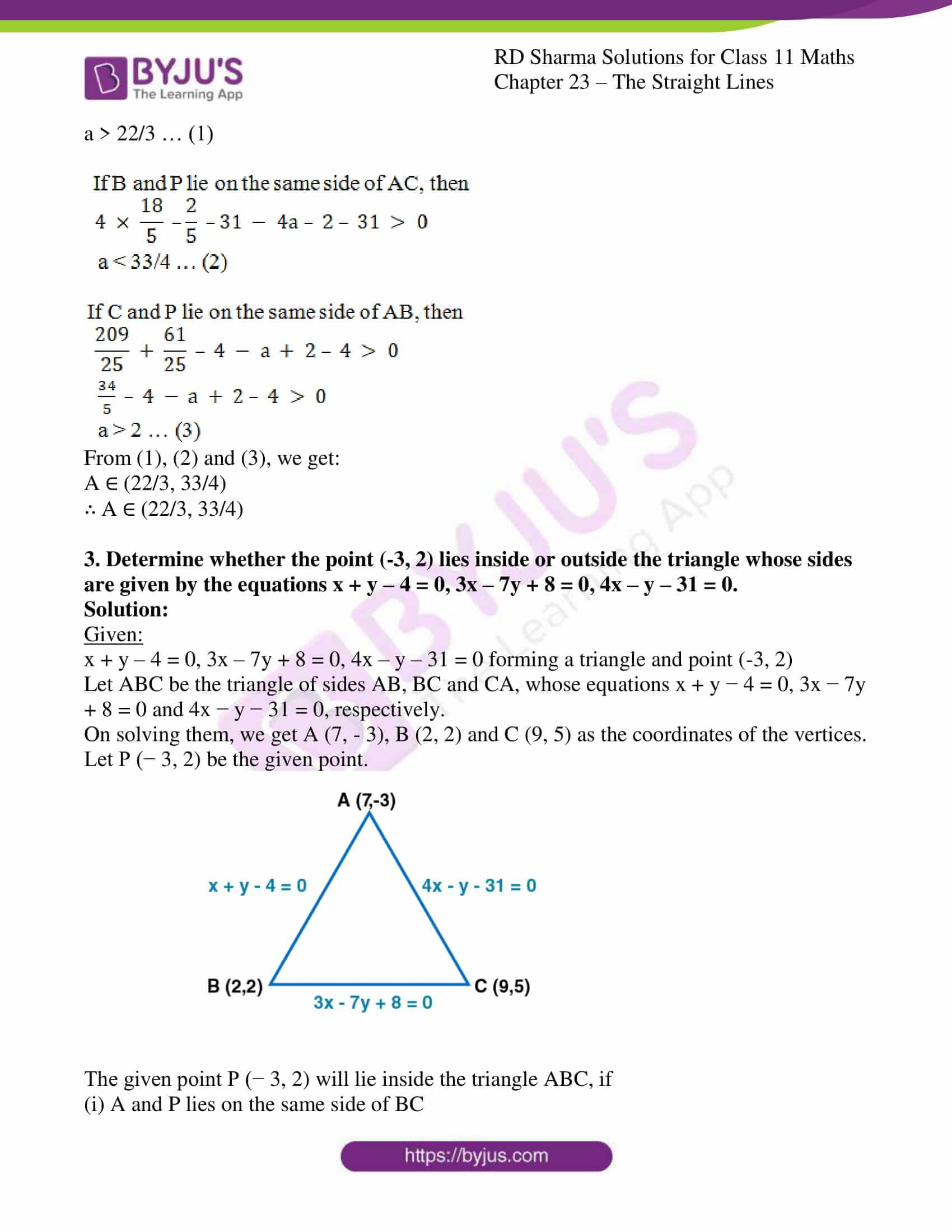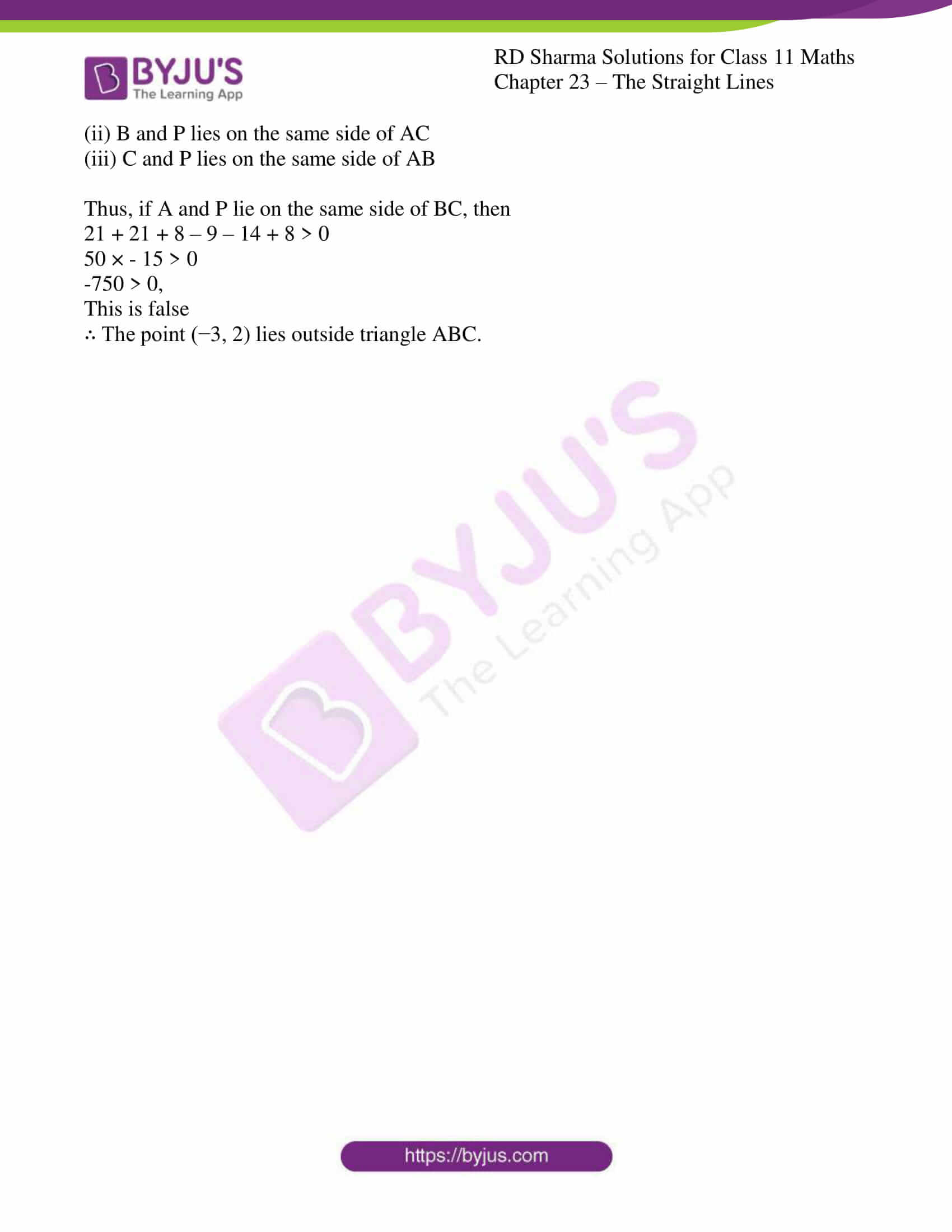### Access answers to RD Sharma Solutions for Class 11 Maths Exercise 23.14 Chapter 23 – The Straight Lines

#### EXERCISE 23.14 PAGE NO: 23.102

1. Find the values of α so that the point P(α 2, α) lies inside or on the triangle formed by the lines x – 5y + 6 = 0, x – 3y + 2 = 0 and x – 2y – 3 = 0.

Solution:

Given:

x – 5y + 6 = 0, x – 3y + 2 = 0 and x – 2y – 3 = 0 forming a triangle and point P(α2, α) lies inside or on the triangle

Let ABC be the triangle of sides AB, BC and CA whose equations are x − 5y + 6 = 0, x − 3y + 2 = 0 and x − 2y − 3 = 0, respectively.

On solving the equations, we get A (9, 3), B (4, 2) and C (13, 5) as the coordinates of the vertices.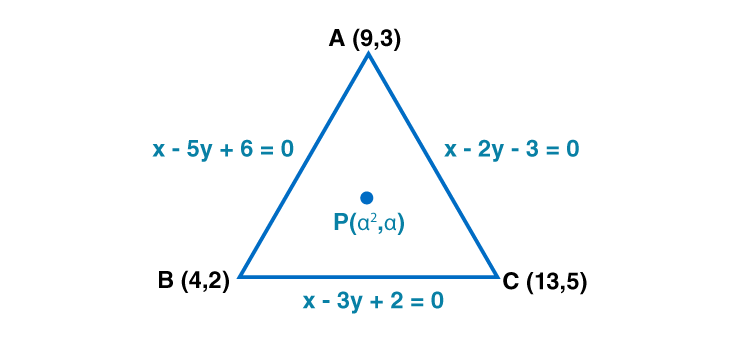It is given that point P (α2, α) lies either inside or on the triangle. The three conditions are given below.

(i) A and P must lie on the same side of BC.

(ii) B and P must lie on the same side of AC.

(iii) C and P must lie on the same side of AB.

If A and P lie on the same side of BC, then

(9 – 9 + 2)(α2 – 3α + 2) ≥0

(α – 2)(α – 1) ≥ 0

α ∈ (- ∞, 1 ] ∪ [ 2, ∞) … (1)

If B and P lie on the same side of AC, then

(4 – 4 – 3) (α2 – 2α – 3) ≥ 0

(α – 3)(α + 1) ≤ 0

α ∈ [- 1, 3] … (2)

If C and P lie on the same side of AB, then

(13 – 25 + 6)(α2 – 5α + 6) ≥0

(α – 3)(α – 2) ≤ 0

α ∈ [ 2, 3] … (3)

From equations (1), (2) and (3), we get

α∈ [2, 3]

∴ α∈ [2, 3]

2. Find the values of the parameter a so that the point (a, 2) is an interior point of the triangle formed by the lines x + y – 4 = 0, 3x – 7y – 8 = 0 and 4x – y – 31 = 0.

Solutions:

Given:

x + y – 4 = 0, 3x – 7y – 8 = 0 and 4x – y – 31 = 0 forming a triangle and point (a, 2)is an interior point of the triangle

Let ABC be the triangle of sides AB, BC and CA whose equations are x + y − 4 = 0, 3x − 7y − 8 = 0 and 4x − y − 31 = 0, respectively.

On solving them, we get A (7, – 3), B (18/5, 2/5) and C (209/25, 61/25) as the coordinates of the vertices.

Let P (a, 2) be the given point.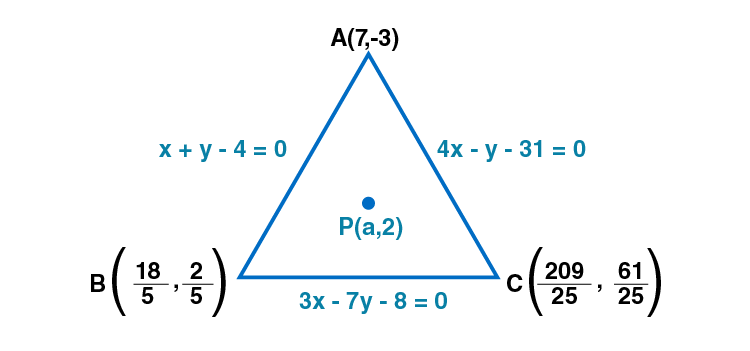It is given that point P (a, 2) lies inside the triangle. So, we have the following:

(i) A and P must lie on the same side of BC.

(ii) B and P must lie on the same side of AC.

(iii) C and P must lie on the same side of AB.

Thus, if A and P lie on the same side of BC, then

21 + 21 – 8 – 3a – 14 – 8 > 0

a > 22/3 … (1)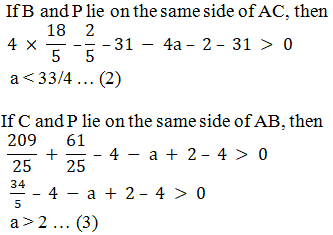From (1), (2) and (3), we get:

A ∈ (22/3, 33/4)

∴ A ∈ (22/3, 33/4)

3. Determine whether the point (-3, 2) lies inside or outside the triangle whose sides are given by the equations x + y – 4 = 0, 3x – 7y + 8 = 0, 4x – y – 31 = 0.

Solution:

Given:

x + y – 4 = 0, 3x – 7y + 8 = 0, 4x – y – 31 = 0 forming a triangle and point (-3, 2)

Let ABC be the triangle of sides AB, BC and CA, whose equations x + y − 4 = 0, 3x − 7y + 8 = 0 and 4x − y − 31 = 0, respectively.

On solving them, we get A (7, – 3), B (2, 2) and C (9, 5) as the coordinates of the vertices.

Let P (− 3, 2) be the given point.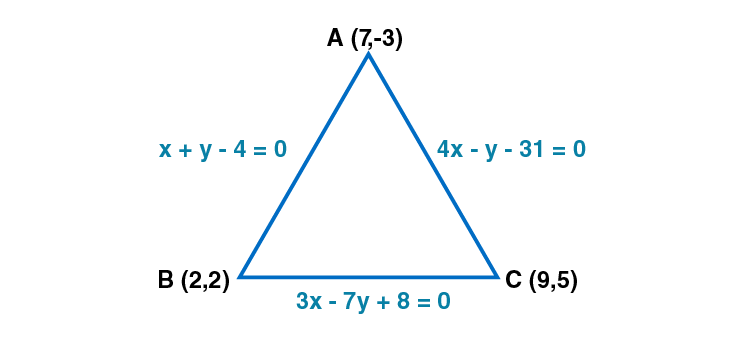The given point P (− 3, 2) will lie inside the triangle ABC, if

(i) A and P lies on the same side of BC

(ii) B and P lies on the same side of AC

(iii) C and P lies on the same side of AB

Thus, if A and P lie on the same side of BC, then

21 + 21 + 8 – 9 – 14 + 8 > 0

50 × – 15 > 0

-750 > 0,

This is false

∴ The point (−3, 2) lies outside triangle ABC.